# H.266/VVC 标准之量化技术技术运营 @ 北京字节跳动科技有限公司

# H.266/VVC 中的量化技术

H.266/VVC 中的量化技术包括均匀标量量化 (Uniform Reconstruction Quantization, URQ)、符号位隐藏 (Sign Data Hiding, SDH)、网格编码量化 (Trellis-coded Quantization, TCQ)。其中 URQ 属于标量量化，而 SDH 和 TCQ 是一种多系数联合量化。URQ 和 SDH 是 HEVC 中已经存在的技术，在 H.266/VVC 中没有进行大的改变。TCQ 是 H.266/VVC 中新引进的量化技术，也称为依赖性量化 (Dependent Quantization, DQ)。

## 均匀标量量化 (URQ)

### URQ 的反量化过程

$\Delta=2^{(QP-4)/6}\cdot 2^{B-8}$

$t'_k=\alpha_k\cdot2^{(QP-4)/6}\cdot 2^{B-8}\cdot q_k$

$t'_k=\alpha_k\cdot2^{(QP-4)/6 + B-8}\cdot 2^{15-B}\cdot (WH)^{-1/2}\cdot q_k$

$p=\lfloor QP/6 \rfloor+B-8$$m=QP\%6$$\beta=\lceil\frac{1}{2}\log_2{WH}\rceil$$\gamma=2\beta-\log_2{WH}$$\lfloor \rfloor$$\lceil \rceil$分别表示向下取整和向上取整，$\%$ 表示取模运算，因为变换块的宽 (W) 和高 (H) 都是 2 的次幂，所以 $\gamma∈{0,1}$，反量化过程可以用如下公式表示：

$t'_k=(16\alpha_k)\cdot(2^{(32+3\gamma+m)/6})\cdot 2^{p}\cdot 2^{5-\beta-B}\cdot q_k$

$t'_k=(w_k(a[\gamma][m]\ll p)\cdot q_k+((1\ll b)\gg 1))\gg b$

$r'_k=((a[m]\ll(p+4))\cdot q_k+512)\gg10$

### URQ 的量化过程

$q_k = sgn(t_k)\cdot \lfloor \frac{|t_k|+f}{\Delta} \rfloor$

$f$ 变大时，量化死区减小；当 $f$ 变小时，量化死区增大。量化死区大小可以直接影响到视频的主观质量。经过变换后，视频中高频部分的数值通常较小，离 0 值较近。如果死区比较大，0 值附近的值会被量化为 0，则视频会损失这些细节。一般情况下，帧内预测时 $f=\Delta/3$，帧间预测时 $f=\Delta/6$

1. 在对变换系数进行量化时，首先使用均匀量化器获取每个变换系数的量化系数，需要比较每个量化系数以及微调后的率失真代价，此时需要考虑每个量化系数的当前值、和当前值减1情况（当前量化系数 0 和 2 除外：当前值为 0，只考虑 0；当前值为 2，考虑 2，1，0），根据不同量化候选值计算率失真代价的大小，为当前位置的系数选择该步骤的量化结果。
2. 在系数组消除的步骤中，编码器计算将整个系数组中的量化系数全部置为 0 时的率失真代价，和第一步中最优的量化结果进行比较，如果此时的率失真代价是最小的，那么该系数组内的全部量化系数置 0。通过仅编码全零系数组标识位而不需要编码系数组的量化系数可以带来可观的码率节省，同时也会带来一定的失真。
3. 在最后一步中，已经遍历完变换块内的所有系数组，需要考虑第一个非零量化系数（解码顺序）的位置，通过计算不同位置的率失真代价，选择最优的第一个非零量化系数的位置。

## 符号位隐藏 (SDH)

H.266/VVC 中的符号位隐藏技术和 HEVC 相比没有变化，SDH 的基本思想是通过在解码端推导出一个系数组的最后一个非零量化系数（解码顺序）的符号，而不用编码这个非零量化系数的符号，从而达到提高压缩效率的目的。和标量量化在相同量化步长的条件下相比，使用 SDH 技术对于每个系数组能够节省一个比特。

### SDH 的编码技术

a) 最后一个非零系数不能调整为 0；

b) 如果第一个非零系数被调整为 0，新的第一个非零系数和最后一个非零系数之间的距离仍然要大于 3；

c) 第一个非零系数到最后一个非零系数之间为 0 的量化系数也可以进行调整，如果它的源信号为正，量化系数可以加 1；如果它的源信号为负，量化系数可以减 1；

d) 量化系数等于 1 时，既可以做加 1 的调整也可以做减 1 的调整；

e) 调整后的量化系数不能超过熵编码时的量化系数取值的最大值或最小值。

## 网格编码量化 (TCQ)

### TCQ 的基本概念

TCQ 的概念最早见于文献，网格编码量化借鉴于网格编码调制 (Trellis-coded Modulation, TCM)。TCQ 通过对信号空间的扩展，提高了量化器的均方误差性能，因而能够以适当的计算复杂度获得比较好的量化性能，是一种对无记忆信源及高斯-马尔科夫（Gauss-Markov）信源比较高效的量化方法。

TCQ 包含若干个标量量化器，在大部分情况下，标量量化器的重构值是量化步长$\Delta$的整数倍。如图 1 所示，TCQ 包含两个标量量化器 $Q_0$$Q_1$，其中$Q_0$能够表示量化步长$\Delta$的所有偶数倍的重构值，$Q_1$ 能够表示量化步长$\Delta$的所有奇数倍的重构值。此外，两个量化器都包含了零倍量化步长（即重构值等于0）的情况，这样的设计能够提高低码率场景下的编码性能。两个量化器的取值分别用量化索引来表示，如在 $Q_0$ 量化器中，量化索引等于3表示的重构值为 $6\Delta$；在 $Q_1$ 量化器中，量化索引等于 -2 表示的重构值为 $-3\Delta$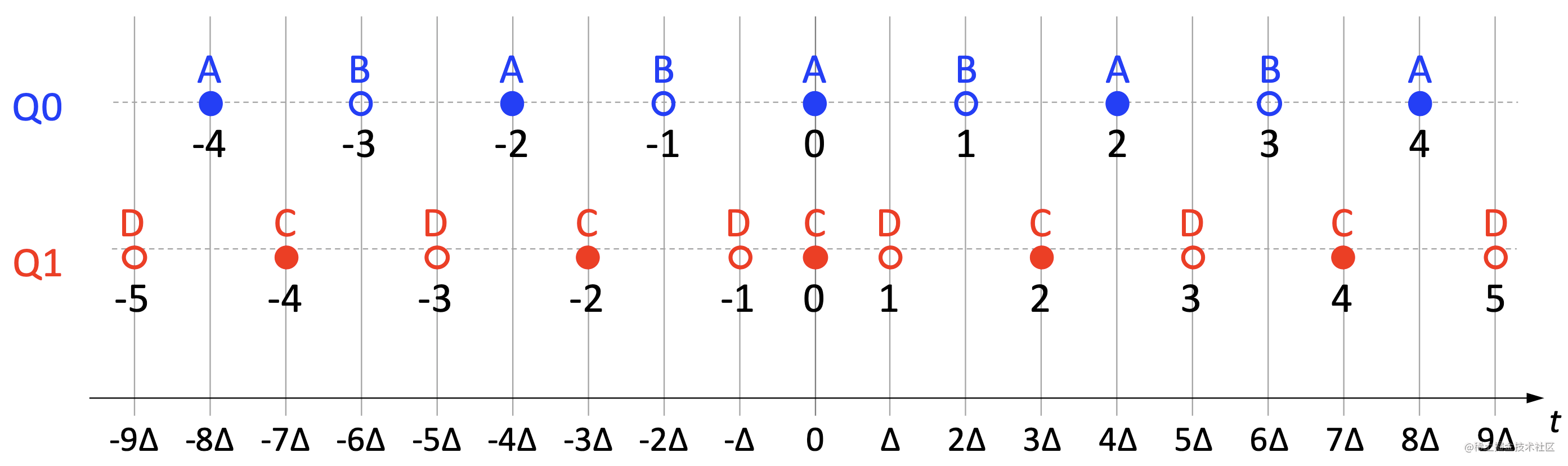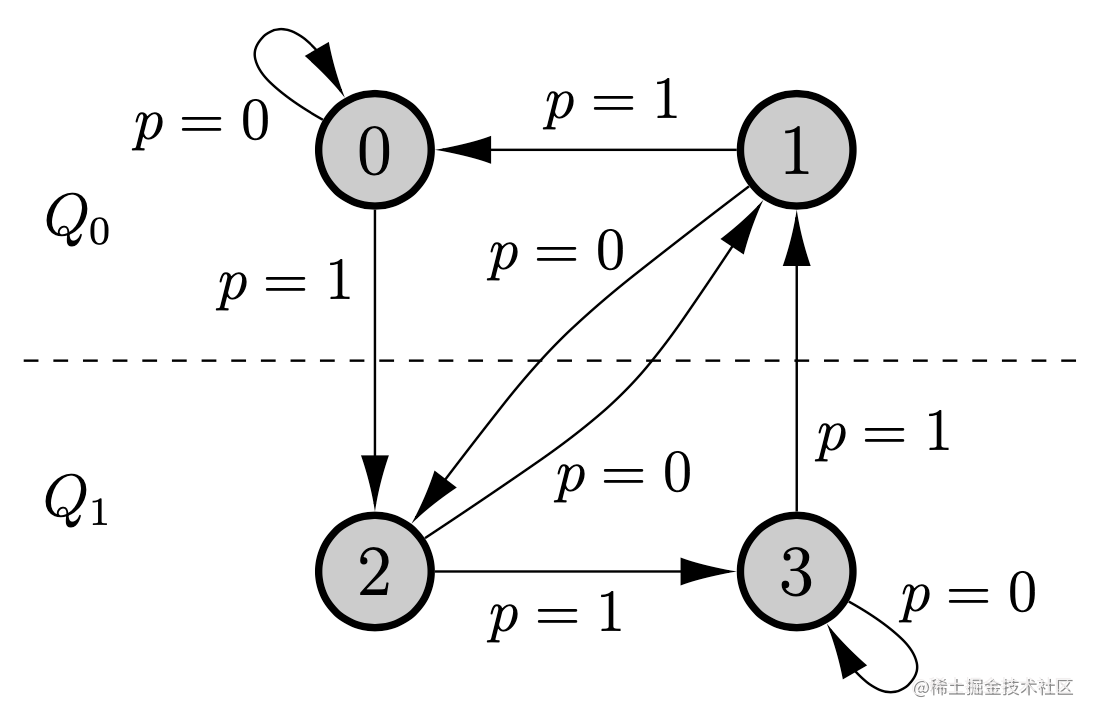### TCQ 的解码过程

$S_0=0$

$for~k=0~to~N-1~do$

$~~t_k'=(2 \cdot q_k-(s_k>>1)\cdot sgn(q_k))\cdot \Delta$

$~~s_{k+1}=stateTransTable[s_k][q_k~\&~1]$

$end~for$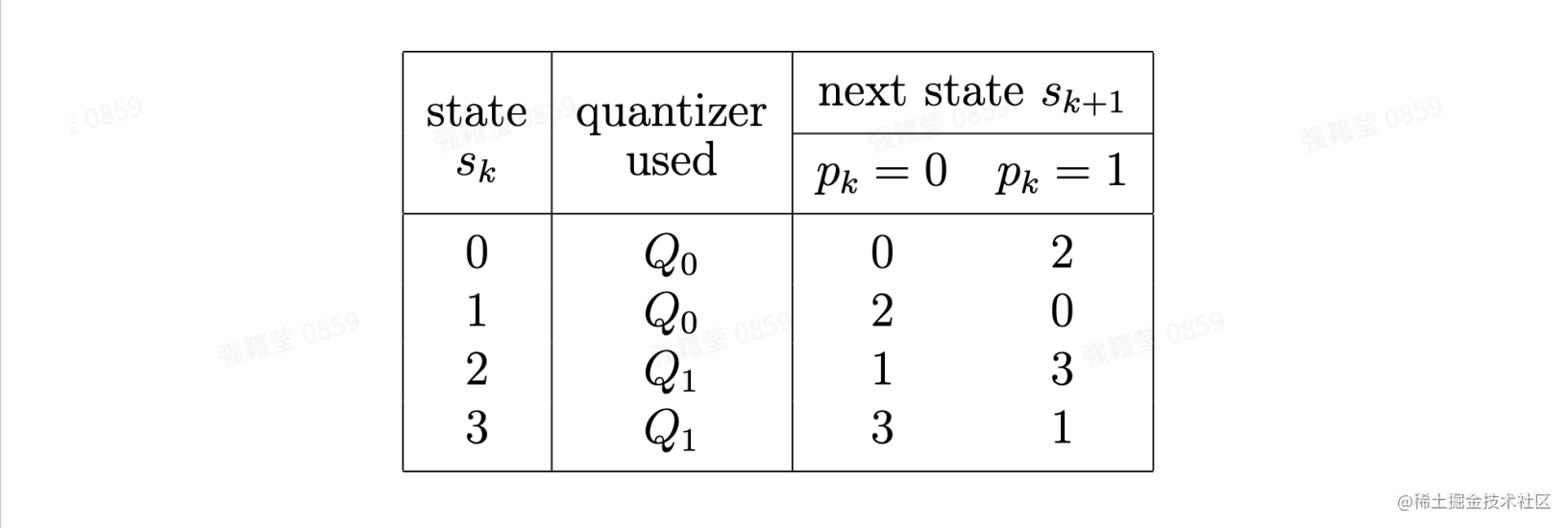图3. 状态转移表

### TCQ 的编码过程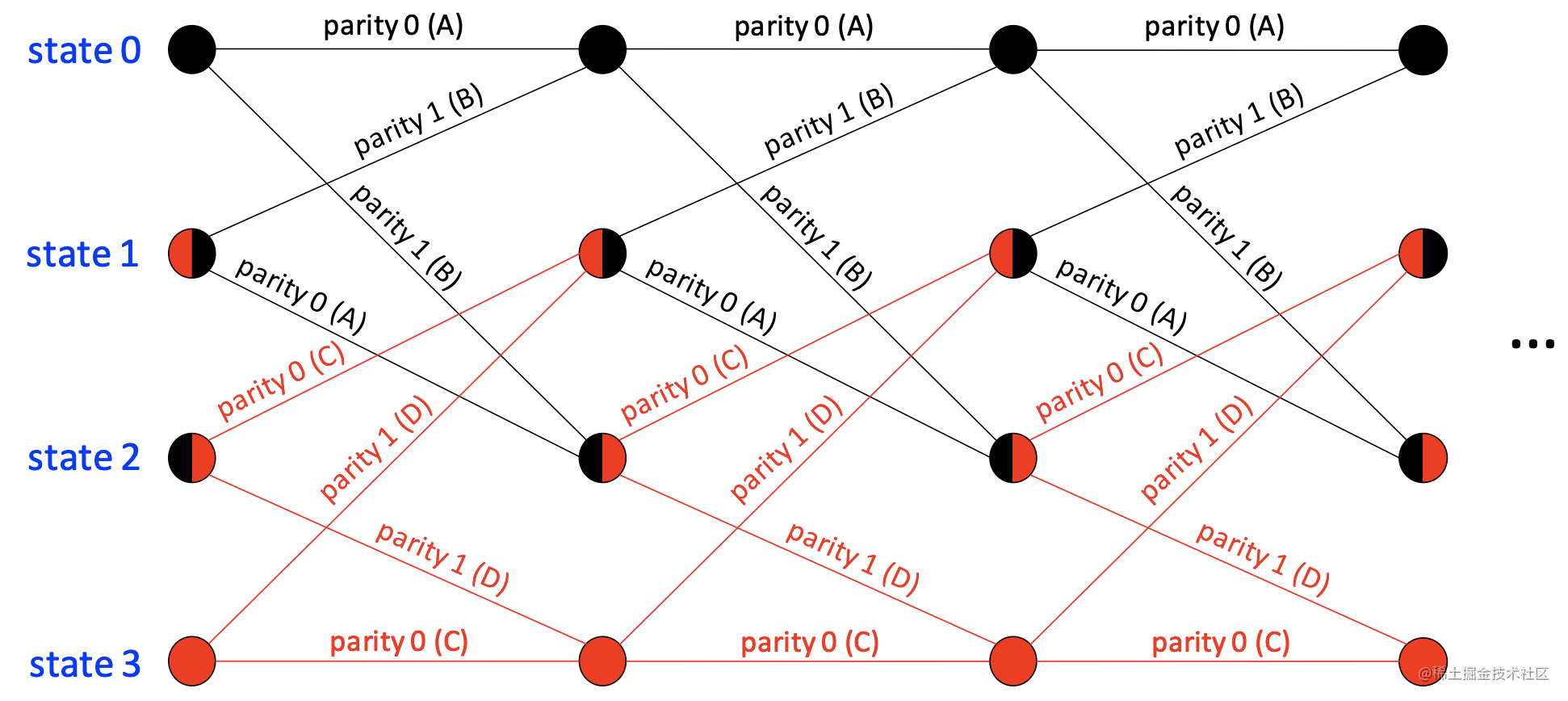图4. 编码端决策量化索引的网格结构

$D_k(q_k, s_k)=(t_k-\Delta \cdot (2 \cdot q_k - (s_k >> 1) \cdot sgn(q_k)))^2$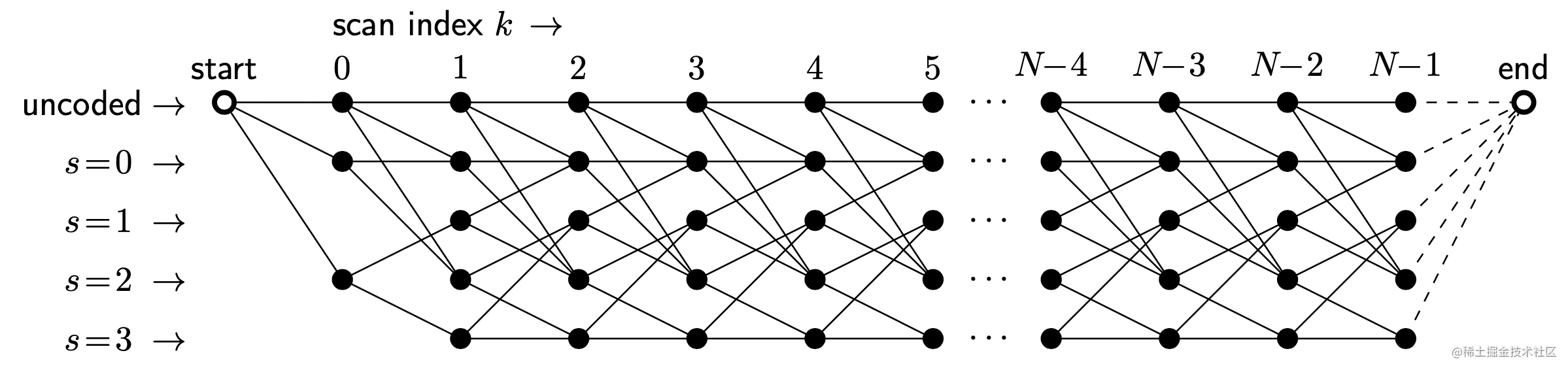图5. 编码端带有“未编码”状态的决策量化索引的网格结构

$uncoded \longmapsto uncoded: J_k=J *{k-1}+D_k(0, 0)$

$uncoded \longmapsto (0, 2): J_k = J*{k - 1} + D_k(q_k, s_k) + \lambda \cdot (R *{first}(x_k, y_k) + R_k(q_k | \cdot \cdot \cdot))$

$(0, 1, 2, 3) \longmapsto (0, 1, 2, 3): J_k = J*{k - 1} + D_k(q_k, s_k) + \lambda \cdot R_k(q_k | \cdot \cdot \cdot)$

## 量化参数控制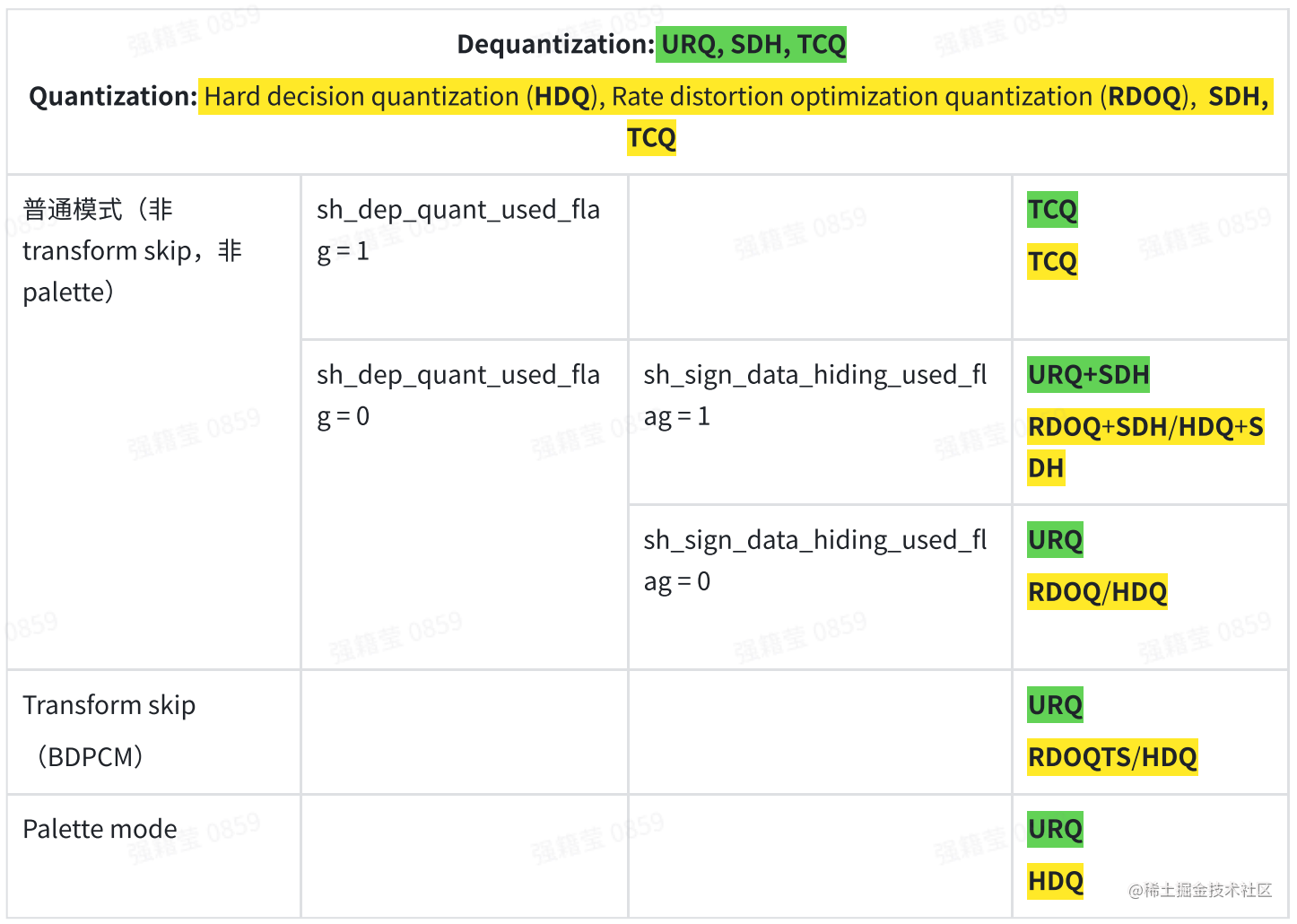H.266/VVC 中用 Scaling list 来表示量化矩阵。对于普通的变换编码模式，Scaling list 中的权重系数默认为 16；对于变换跳过模式，由于不存在反变换过程，所以它的量化过程不受 Scaling list 影响。H.266/VVC 包括 28 个 Scaling lists，每一个 Scaling list 定义了 2x2、4x4、8x8 的权重矩阵。Scaling list 可以在高层语法结构 Adaption parameter set（APS） 中传输，允许直接复用之前的 Scaling list 以及 Scaling list 每个权重系数的差分编码。Scaling list 的选择由变换块的颜色分量、预测模式、宽和高的最大值共同决定，对于大小不等于 2x2、4x4、8x8 的块，权重矩阵通过使用最近邻插值重采样得到。

# 参考文献

 H. Schwarz, M. Coban, M. Karczewicz, T.-D. Chuang, F. Bossen, A. Alshin, J. Lainema, C. R. Helmrich and T. Wiegand, "Quantization and Entropy Coding in the Versatile Video Coding (VVC) Standard," in IEEE Transactions on Circuits and Systems for Video Technology, doi: 10.1109/TCSVT.2021.3072202.

 G.J. Sullivan, "On embedded scalar quantization", IEEE International Conference on Acoustics, Speech, and Signal Processing, ICASSP 2004, vol.4, pp. 605-608, Montreal, Canada, May 2004.

 J.-R. Ohm, G.J. Sullivan, H. Schwarz, T.K. Tan; T. Wiegand, "Comparison of the Coding Efficiency of Video Coding Standards— Including High Efficiency Video Coding (HEVC)," IEEE Transactions on Circuits and Systems for Video Technology, vol. 22, no. 12, pp. 1669-1684, Dec. 2012.

 G. Clare, F. Henry, and J. Jung, Sign Data Hiding, JCTVC-G271, 7th Joint Collaborative Team on Video Coding (JCT-VC) Meeting, Geneva, Switzerland, Nov. 2011.

 X. Yu, J. Wang, D. He, G. Martin-Cocher, and S. Campbell, Multiple Sign Bits Hiding, JCTVC-H0481, 8th Joint Collaborative Team on Video Coding (JCT-VC) Meeting, San Jose, CA, Feb. 2012.

 M. W. Marcellin and T. R. Fischer, “Trellis coded quantization of memoryless and gauss-markov sources,” IEEE Transactions on Communications, vol. 38, no. 1, pp. 82–93, Jan. 1990.

 H. Schwarz, T. Nguyen, D. Marpe and T. Wiegand, "CE7: Transform Coefficient Coding and Dependent Quantization (Tests 7.1.2, 7.2.1)," JVET-K0071, 2018.

 H. Schwarz, T. Nguyen, D. Marpe, and T. Wiegand, “Hybrid video coding with trellis-coded quantization,” in 2019 Data Compression Conference (DCC), March 2019, pp. 182–191.

 G. D. Forney, Jr., “The Viterbi algorithm,” Proceedings of the IEEE, vol. 61, no. 3, pp.

268–278, Mar. 1973.

 M. Wang, S. Wang, J. Li, L. Zhang, Y. Wang, S. Ma and S. Kwong, "Low Complexity Trellis-Coded Quantization in Versatile Video Coding," in IEEE Transactions on Image Processing, vol. 30, pp. 2378-2393, 2021, doi: 10.1109/TIP.2021.3051460.